/

### The logarithmic distribution

The logarithmic distribution arises from following power series expansion: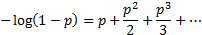This means that the function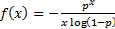,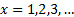can naturally be interpreted as a probability mass function since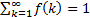.

 Distribution name Logarithmic distribution Common notation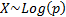Parameters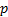= shape parameter (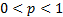) Domain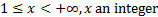Probability mass function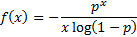Cumulative distribution function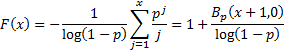Mean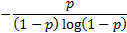Variance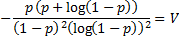Skewness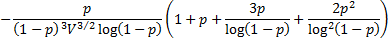(Excess) kurtosis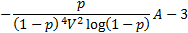where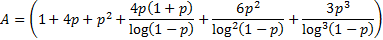Characteristic function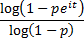Other comments The logarithmic distribution has a mode  of 1. If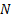is a random variable with Poission distribution and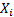,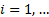is an infinite sequence of iid random variables each distributed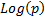then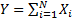has a negative binomial distribution showing that the negative binomial distribution is an example of a compound Poisson distribution

Nematrian web functions

Functions relating to the above distribution may be accessed via the Nematrian web function library by using a DistributionName of “logarithmic”. For details of other supported probability distributions see here.